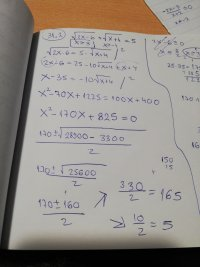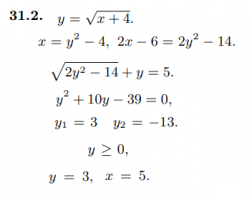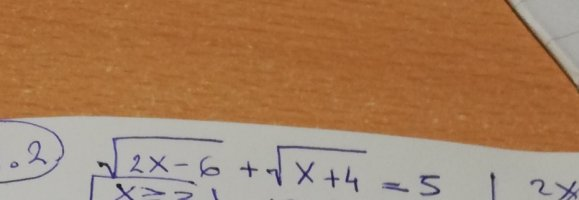How is the answer 165 excluded?

Loki123

Junior Member
The first picture is the problem and how I solved it. I got x=>3 as the domain because of the square roots. In the end I got the answers 5 and 165. The second picture is how the solution actually is. They replaced sqrt(x+4) with y which I did not. In the end they had two answers for y and were able, because of the condition, to discard one and in the end only got x=5. Is there a way for me to discard 165 without going back to check the answers. For instance when I put 165 as x I get 18+13, it would work if it was -13 but sqrt can only be positive (if i am not mistaken in this case)Jomo

Elite Member
sqrt(25) = 5 so nothing under the sqrt signs should be bigger than 25. OK?

Loki123

Junior Member
sqrt(25) = 5 so nothing under the sqrt signs should be bigger than 25. OK?
I am not sure I understand why.

Otis

Elite Member
Is there a way for me to discard 165 without going back to check the answers.
Hi Loki. We must always check answers obtained by squaring both sides of an equation because squaring can eliminate negative signs.

You'd correctly found that the domain of x is all numbers greater than or equal to 3. Therefore, both of your x-values 5 and 165 are valid candidates for solution.

The next step is to find corresponding y-values. Use the first given equation for that. You will get four (x,y) pairs. Two of those pairs may be eliminated because the first given equation tells us that y must be greater than or equal to zero.

Check the remaining two pairs using the following equation, and you'll find that only one of them works.

sqrt(2y^2 - 14) + y = 5

sqrt can only be positive
The square root of a Real number must be positive or zero.Loki123

Junior Member
Hi Loki. We must always check answers obtained by squaring both sides of an equation because squaring can eliminate negative signs.

You'd correctly found that the domain of x is all numbers greater than or equal to 3. Therefore, both of your x-values 5 and 165 are valid candidates for solution.

The next step is to find corresponding y-values. Use the first given equation for that. You will get four (x,y) pairs. Two of those pairs may be eliminated because the first given equation tells us that y must be greater than or equal to zero.

Check the remaining two pairs using the following equation, and you'll find that only one of them works.

sqrt(2y^2 - 14) + y = 5

The square root of a Real number must be positive or zero.I am confused. Where did y come from???

Jomo

Elite Member
I am not sure I understand why.
The right hand side is 5. The left hand side is the sum of square roots so each term is zero or more. If any of the square root term is more than 5, then the how left hand side will be more than 5.
So sqrt(2x-6)<= 5 and sqrt(x+4)<=5

That is 2x-6<=25 or x<=31/2 and x+4<=25 or x<=21. So x<=31/2

Otis

Elite Member
I am confused. Where did y come from???
Are you asking about potential y-values corresponding to x=5 and x=165? If so, you need to find them.

From the first given equation, you know that y is sqrt(x+4). Use substitution (x=5 and x=165) and evaluate.Loki123

Junior Member
The right hand side is 5. The left hand side is the sum of square roots so each term is zero or more. If any of the square root term is more than 5, then the how left hand side will be more than 5.
So sqrt(2x-6)<= 5 and sqrt(x+4)<=5

That is 2x-6<=25 or x<=31/2 and x+4<=25 or x<=21. So x<=31/2
Okay, I understand now how you got that, however, I am not sure why we have to set those conditions. I understand that they have to equal 5 combined so thats why, but why won't solving the equation normally take that into account on its own. For instance if I have (2x+2)+(3x+1)=13 I am not going to write 2x+2<=13 x<=11/2 or 3x+1<=13 x<=4...
Technically those conditions are true, but there is no reason for them... So why use them now? And don't say "it's because they are sqrts" because I still don't understand how that does anything...

Loki123

Junior Member
Are you asking about potential y-values corresponding to x=5 and x=165? If so, you need to find them.

From the first given equation, you know that y is sqrt(x+4). Use substitution (x=5 and x=165) and evaluate.I didn't use y. The second picture is the solution and the first one is my attempt. I didn't use y because frankly I don't see a reason to. If you are implying I need to insert a function y, please be more elaborate because so far I am lost.

Otis

Elite Member
The second picture is the solution and the first one is my attempt
Yes, that is obvious. Your work shows that you got to candidates x=5, x=165 and then stopped.

What y values correspond to those potential x-values?

x = y^2 - 4

Substitute x=5 and solve for y. That yields candidates (5,3) and (5,-3).

Substitute x=165 and solve for y. That yields candidates (165,13) and (165,-13).

Check to see which candidates work in all given equations.

PS: You showed the solution, but you'd never posted the exercise. I had to guess which info in the solution was given in the exercise statement. Maybe I've guessed incorrectly. In future threads, please post exercise statements as given.Loki123

Junior Member
Yes, that is obvious. Your work shows that you got to candidates x=5, x=165 and then stopped.

What y values correspond to those potential x-values?

x = y^2 - 4

Substitute x=5 and solve for y. That yields candidates (5,3) and (5,-3).

Substitute x=165 and solve for y. That yields candidates (165,13) and (165,-13).

Check to see which candidates work in all given equations.

PS: You showed the solution, but you'd never posted the exercise. I had to guess which info in the solution was given in the exercise statement. Maybe I've guessed incorrectly. In future threads, please post exercise statements as given.It's this one.I am sorry, but I don't understand why we need y values.

Dr.Peterson

Elite Member
I didn't use y. The second picture is the solution and the first one is my attempt. I didn't use y because frankly I don't see a reason to. If you are implying I need to insert a function y, please be more elaborate because so far I am lost.

I am sorry, but I don't understand why we need y values.
It is not necessary to use y. That is just what they chose to do. There are multiple ways to solve any interesting problem.

I would have done exactly what you did, because that is how textbooks I've taught from do it. Their method gave them a way to eliminate an extraneous solution; but I'm not sure it guarantees that any solution they don't eliminate is a valid one. Ultimately, I think you still need to check. So they are giving you an answer to your question,
Is there a way for me to discard 165 without going back to check the answers.
But even they haven't (in what you showed) promised that you never have to check.

For instance when I put 165 as x I get 18+13, it would work if it was -13 but sqrt can only be positive
This is a good observation. What is happening in these problems is that each time you square, you are losing information about a sign; so in your work you are actually solving four equations simultaneously: [imath]\pm\sqrt{2x-6} \pm \sqrt{x+4}=5[/imath].

It happens that 5 was the solution of [imath]+\sqrt{2x-6} + \sqrt{x+4}=5[/imath], and 165 was the solution of [imath]\sqrt{2x-6} - \sqrt{x+4}=5[/imath]. (The other two equations, with -+ and --, have no solutions.) I suspect that if they had let [imath]y=\sqrt{2x-6}[/imath], they might not have caught the extraneous solution, though I haven't bothered to try.

Otis

Elite Member
I don't understand why we need y values.
We don't need to introduce any new variables. While guessing (from your image) what they'd given you, I'd thought some of the equations involving y had been provided in the exercise statement.•Loki123

Otis

Elite Member
How is the answer 165 excluded?
165 is not an answer. It does not satisfy the given equation.

[imath]\;[/imath]

•Loki123

Loki123

Junior Member
I suspect that if they had let y=2x−6y=\sqrt{2x-6}y=2x−6, they might not have caught the extraneous solution, though I haven't bothered to try.
I just tried it. You get 165 and 5 that way too.

Loki123

Junior Member
165 is not an answer. It does not satisfy the given equation.

[imath]\;[/imath]
I thought that I missed some condition and that that was the reason i got two answers.

Otis

Elite Member
I thought that I missed some condition and that that was the reason i got two answers.
You did not get two answers. There is only one answer. (You'd gotten two results, which I call "candidates".)

We often get extraneous solutions, after having squared an equation. That is why we need to check all results.

[imath]\;[/imath]

Jomo

Elite Member
Okay, I understand now how you got that, however, I am not sure why we have to set those conditions. I understand that they have to equal 5 combined so thats why, but why won't solving the equation normally take that into account on its own. For instance if I have (2x+2)+(3x+1)=13 I am not going to write 2x+2<=13 x<=11/2 or 3x+1<=13 x<=4...
Technically those conditions are true, but there is no reason for them... So why use them now? And don't say "it's because they are sqrts" because I still don't understand how that does anything...
You can add a very positive number to some number to sum to 5. You can add a very negative number to a number to sum to 5. In fact, the line x+y = 5 shows this.
However, your problems does not allow this! The terms that you are adding are both non-negative. This restricts both terms to between 0 and 5 inclusive. Do you see this?
Basically you need to understand how to graph |x| + |y| = 5. What value can |x| be? How about |y|? Note that I did not ask what x and y can be!

Jomo

Elite Member
Okay, I understand now how you got that, however, I am not sure why we have to set those conditions. I understand that they have to equal 5 combined so thats why, but why won't solving the equation normally take that into account on its own. For instance if I have (2x+2)+(3x+1)=13 I am not going to write 2x+2<=13 x<=11/2 or 3x+1<=13 x<=4...
Technically those conditions are true, but there is no reason for them... So why use them now? And don't say "it's because they are sqrts" because I still don't understand how that does anything...
NO, there is no reason at all why 2x+2 <=13! 2x+2 can equal 113 as long as 3x+1 = -100.
There is no reason at all to assume that both 2x+2 and 3x+1 are both positive or 0!
However, in your problem both terms MUST be positive or 0. You can't take the sqrt of a number and get less than 0. Don't you see the difference? You say that you understand but then give an example which shows you do not understand.

Harry_the_cat

Elite Member
Rather than worry about the domain, I think it is much simpler to check your "answers" by substituting back into original equation and see if they work. Clearly one of them doesn't. Checking your answers this way is a good habit anyway.

•Subhotosh Khan, Jomo and Otis